JEE  >  Test: Previous Year Questions - Sequences And Series- 2

# Test: Previous Year Questions - Sequences And Series- 2

Test Description

## 18 Questions MCQ Test Mathematics (Maths) Class 11 | Test: Previous Year Questions - Sequences And Series- 2

Test: Previous Year Questions - Sequences And Series- 2 for JEE 2023 is part of Mathematics (Maths) Class 11 preparation. The Test: Previous Year Questions - Sequences And Series- 2 questions and answers have been prepared according to the JEE exam syllabus.The Test: Previous Year Questions - Sequences And Series- 2 MCQs are made for JEE 2023 Exam. Find important definitions, questions, notes, meanings, examples, exercises, MCQs and online tests for Test: Previous Year Questions - Sequences And Series- 2 below.
Solutions of Test: Previous Year Questions - Sequences And Series- 2 questions in English are available as part of our Mathematics (Maths) Class 11 for JEE & Test: Previous Year Questions - Sequences And Series- 2 solutions in Hindi for Mathematics (Maths) Class 11 course. Download more important topics, notes, lectures and mock test series for JEE Exam by signing up for free. Attempt Test: Previous Year Questions - Sequences And Series- 2 | 18 questions in 18 minutes | Mock test for JEE preparation | Free important questions MCQ to study Mathematics (Maths) Class 11 for JEE Exam | Download free PDF with solutions
 1 Crore+ students have signed up on EduRev. Have you?
Test: Previous Year Questions - Sequences And Series- 2 - Question 1

### (a) Consider an infinite geometric series with first term a and common ratio r. If the sum is 4 and the second term is 3/4, then        [JEE 2000, (scr.), 1 + 1] (A) a =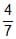, r =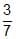(B) a = 2, r =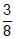(C) a = , r =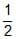(D) a = 3, r =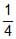(b) If a, b, c, d are positive real numbers such that a + b + c + d = 2, then M = (a + b) (c + d) satisifes the relation : (A) 0 £ M £ 1 (B) 1 £ M £ 2 (C) 2 £ M £ 3 (D) 3 £ M £ 4 (c) The fourth power of the common difference of an arithmetic progression with integer entries added to the product of any four consecutive terms of it. Prove that the resulting sum is the square of an integer. [JEE 2000, (Mains), 4]

Test: Previous Year Questions - Sequences And Series- 2 - Question 2

### Given that a, g are roots of the equation, Ax2_4x+1 =0 and b, d the roots of the equation, Bx2 _ 6x + 1 = 0, find values of A and B, such that a, b, g & d are in H.P.       [REE 2000, 5]

Test: Previous Year Questions - Sequences And Series- 2 - Question 3

### The sum of roots of the equation ax2 + bx + c = 0 is equal to the sum of squares of their reciprocals. Find whether bc2, ca2 and ab2 in A.P., G.P. or H.P. ?       [ree 2001, 3]

Test: Previous Year Questions - Sequences And Series- 2 - Question 4

Solve the following equations for x and y log2x + log4x + log16x +..............

........... = y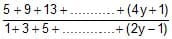= 4log4 x     [ree 2001, 5]

Test: Previous Year Questions - Sequences And Series- 2 - Question 5

a) Le a, b be the roots of x2 _ x + p = 0 and g, d the roots of x2 _ 4x + q = 0. If a, b, g, d are in G.P., then the integral values of p and q respectively, are

(A) _2, _32 (B) _2, 3 (C) _6, 3 (D) _6. _ 32

(b) If the sum of the first 2n terms of the A.P. 2, 5, 8,... ..........is equal to the sum of the first n terms of the A.P. 57, 59, 61,......., then n equals

(A) 10 (B) 12 (C) 11 (D) 13

(c) Let the positive numbers a, b, c, d be in A.P. Then abc, abd, acd, bcd are [Jee 2001, (Scr.) 1 + 1 + 1]

(A) NOT in A.P./G.P./ H.P. (B) in A.P. (C) in G.P. (D) in H.P.

(d) Let a1, a2,..... be positive real numbers in G.P. For each n, let An, Gn, Hn be respectively, the arithmetic mean, geometric mean, and harmonic mean of a1, a2,..........., an. Find an expression for the G.M. of G1, G2,............, Gn in terms of A1, A2,...........,An, H1, H2,........,Hn.                 [JEE 2001 (Mains), 5]

Test: Previous Year Questions - Sequences And Series- 2 - Question 6

(a) Suppose a, b, c are in A.P. and a2, b2, c2 are in G.P. if a < b < c and a + b + c = 3/2, then the value of a is [JEE 2002 (Scr.), 3]

(A)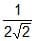(B)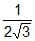(C)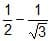(D)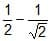(b) Let a, b be positive real numbers. If a, A1, A2,b are in A.P. ; a, G1, G2, b are in G.P. and a, H1, H2, b are in H.P. show that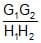=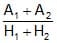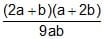.                    [JEE 2002 (Mains), 5]

Test: Previous Year Questions - Sequences And Series- 2 - Question 7

The first term of an infinite geometric progression is x and its sum is 5. Then         [JEE 2004 (Scr.)]

Test: Previous Year Questions - Sequences And Series- 2 - Question 8

(a) In the quadratic equation ax2 + bx + c = 0, If D = b2 _ 4ac and a + b, a2 + b2, a3+ b3, are in G.P. where a, b are the roots of ax2 + bx + c = 0, then [JEE 2005 (Scr.)]

(A) D ¹ 0 (B) bD = 0 (C) cD = 0 (D) D = 0

(b) If total number of runs scored in n matches is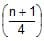(2n+1 _ n _ 2) where n > 1, and the runs scored in the kth match are given by k.2n+1_k, where 1 £ k £ n. Find n.               [JEE 2005 (Mains), 2]

Test: Previous Year Questions - Sequences And Series- 2 - Question 9

If An =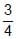_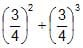+ ............(_1)n _ 1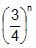and Bn = 1 _ An, then find the minimum natural number n0 such that Bn > An n > n0.           [JEE 2006, 6]

Test: Previous Year Questions - Sequences And Series- 2 - Question 10

Let Vr denote the sum of first r terms of an arithmetic progression (A.P.) whose first term is r and the common difference is (2r _ 1). Let Tr = V r + 1 _ Vr _ 2 and Qr= Tr + 1 _ Tr for r = 1, 2,.....

(a) The sum V1 + V2 + ....... + Vn is

(A)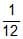n(n+1)(3n2 _n+1) (B)n(n+1)(3n2+n+2) (C)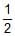n (2n2_n+1) (D)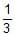(2n3_2n+3)

(b) Tr is always

(A) an odd number (B) an even number (C) a prime number (D) a composite number

(c) Which one of the following is a correct statement ?

(A) Q1, Q2, Q3,.... are in A.P., with common difference 5

(B) Q1, Q2, Q3,.... are in A.P., with common difference 6

(C) Q1, Q2, Q3,.... are in A.P., with common difference 11

(D) Q= Q= Q=....                                                        [JEE 2007, 4 + 4 + 4]

Test: Previous Year Questions - Sequences And Series- 2 - Question 11

Let A1, G1, H1 denote the arithmetic, geometric and harmonic means, respectively, of two distinct positive numbers. For n ³ 2, Let An _ 1 and Hn _ 1 have arithmetic, geometric and harmonic means as An, Gn, Hn respectively

(a) Which one of the following statements is correct ?

(A) G1 > G2 > G3 > ........ (B) G1 < G2 < G3 < ........

(C) G1 = G2 = G3 = ........ (D) G1 < G3 < G5 < ...... and G2 > G4 > G6 > ......

(b) Which one of the following statement is correct ?

(A) A1 > A2 > A3 > ........ (B) A1 < A2 < A3 < ........

(C) A1 > A3 > A5 > .....and A2 < A4 < A6 < ........ (D) A1 < A3 < A5 < .....and A2 > A4 > A6 > ........

(c) Which one of the following statement is correct ?            [JEE 2007, 4 + 4 + 4]

(A) H1 > H2 > H3 > ........ (B) H1 < H2 < H3 < ........

(C) H1 > H3 > H5 > .....and H2 < H4 < H6 < ........ (D) H1 < H3 < H5 < .....and H2 > H4 > H6 > ........

Test: Previous Year Questions - Sequences And Series- 2 - Question 12

(a) A straight line through the vertex P of a triangle PQR intersects the side QR at the point S and the circumcircle of the triangle PQR at the point T. If S is not the centre of the circumcircle, then     [JEE 2008, 4]

(A)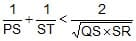(B)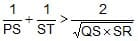(C)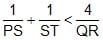(D)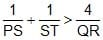(b) Supose four distinct positive numbers a1, a2, a3, a4 are in G.P. Let b1 = a1, b2 = b1 + a2, b3 = b2 + a3 and b4 = b3 + a4. [JEE 2008, 3]

Statement_1 : The numbers b1, b2, b3, b4 are neither in A.P. nor in G.P.

Statement_2 : The numbers b1, b2, b3, b4 are in H.P.

(A) Statement (1) is true and statement (2) is true and statement (2) is correct explanation for (1)

(B) Statement (1) is true and statement (2) is true and statement (2) is NOT correct explanation for (1)

(C) Statement (1) is true but (2) is false

(D) Statement (1) is false but (2) is true

Test: Previous Year Questions - Sequences And Series- 2 - Question 13

If the sum of first n terms of an A.P. is cn2, then the sum of squares of these n terms is    [JEE 2009, 3]

Detailed Solution for Test: Previous Year Questions - Sequences And Series- 2 - Question 13

Sn = cn2
S(n−1) = c(n−1)2
= cn2+c−2cn
Tn = 2cn − c
Tn2 = (2cn−c)2
= 4c2n2 + c2 − 4c2 n
Required sum = ∑Tn2 = 4c2 ∑n2 +nc2 −4c2 ∑n
{4c2n(n+1)(2n+1)}/6 + nc2−2c2n(n+1)
= (2c2n(n+1)(2n+1)+3nc2−6c2n(n+1))/3
​= {nc2[4n2+6n+2+3−6n−6]}/3
​= [nc2(4n2−1)]/3

*Answer can only contain numeric values
Test: Previous Year Questions - Sequences And Series- 2 - Question 14

Let Sk, K = 1, 2, ...., 100 denote the sum of the infinite geometric series whose first term is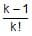and the common ratio is 1/k. Then the value of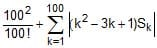is        [JEE 2010]

Detailed Solution for Test: Previous Year Questions - Sequences And Series- 2 - Question 14

Infinite Sum of GP S = a/(1−r) = (k−1)/[k!(1−1/k)] = 1/(k−1)!
​(100)2/100! + ∑k=(1 to 100)|(k2−3k+1)Sk|
(100)2/100! + ∑k=(1 to 100) [(k-1)^2 k[(1/(k-1)!]
(100)2/100! + ∑k=(2 to 100)|[(k-1)/(k-2)! - k/(k-1)!|
(100)2/100! 2/1! - 1/0 + 2/1! - 3/2! + 3/2! +.....+ 99/98! - 100/99!
= (100)2/100! + 2 - 1 + 2 - dfrac{100 * 100}{99! * 100}
= 3

*Answer can only contain numeric values
Test: Previous Year Questions - Sequences And Series- 2 - Question 15

Let a1, a2, a3, ....., a11 be real numbers satisfying

a1 = 15, 27 _ 2a2 > 0 and ak = 2ak-1 _ ak-2 for k = 3, 4, ...., 11

If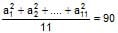, then the value of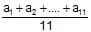is equal to        [JEE 2010]

*Answer can only contain numeric values
Test: Previous Year Questions - Sequences And Series- 2 - Question 16

The minimum value of the sum of real numbers a_5, a_4, 3a_3, 1, a8 and a10 with a > 0 is    [JEE 2011]

*Answer can only contain numeric values
Test: Previous Year Questions - Sequences And Series- 2 - Question 17

Let a1, a2, a3, ..., a100 be an arithmetic progression with a1 = 3 and Sp =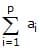, 1 £ p £ 100. For any integer n with 1 £ n £ 20. let m=5n. If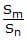does not depend on n, then a2 is     [JEE 2011]

Test: Previous Year Questions - Sequences And Series- 2 - Question 18

Let a1, a2, a3, ..... be in harmonic progression with a1 = 5 and a20 = 25. The least positive integer n for which an < 0 is         [JEE 2012]

Detailed Solution for Test: Previous Year Questions - Sequences And Series- 2 - Question 18## Mathematics (Maths) Class 11

156 videos|176 docs|132 tests
 Use Code STAYHOME200 and get INR 200 additional OFF Use Coupon Code
Information about Test: Previous Year Questions - Sequences And Series- 2 Page
In this test you can find the Exam questions for Test: Previous Year Questions - Sequences And Series- 2 solved & explained in the simplest way possible. Besides giving Questions and answers for Test: Previous Year Questions - Sequences And Series- 2, EduRev gives you an ample number of Online tests for practice

## Mathematics (Maths) Class 11

156 videos|176 docs|132 tests